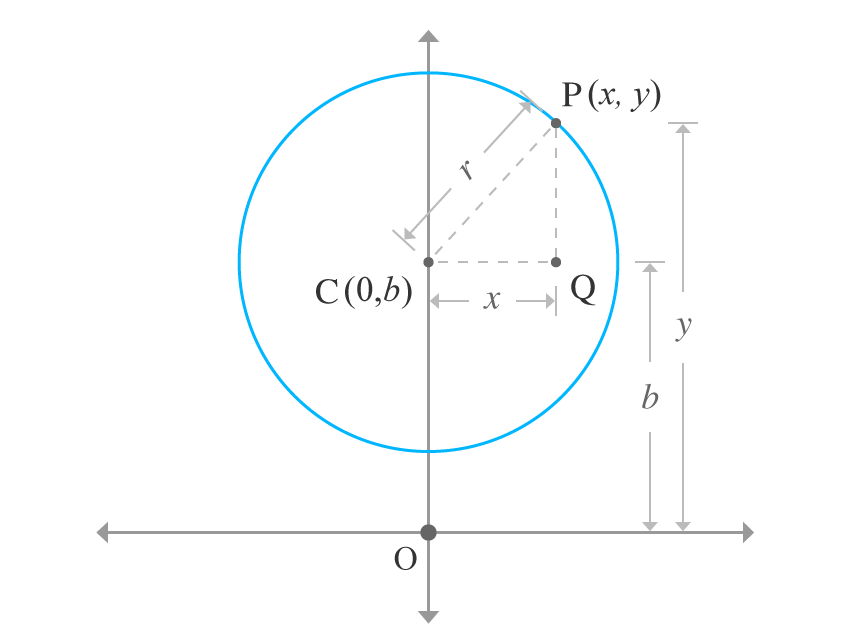# Equation of a circle when centre of the circle lies on y-axis

## Equation

$x^2 + {(y-b)}^2 = r^2$
$x^2 + y^2 -2by + b^2 -r^2 = 0$

### Proof

Consider a circle having radius of $r$ units in two dimensional Cartesian coordinate system. The centre of circle lies on $y$-axis without touching the $x$-axis. The centre of the circle is $b$ units distance from the origin. Therefore, the centre of circle is $C(0, b)$. Take $P$ as a point on the circumference of the circle and its coordinates are $x$ and $y$. Therefore, the point is $P(x, y)$.Draw a line from point $C$ and it is parallel to horizontal axis and also draw another straight line from point $P$ and it should be parallel to vertical axis. The two lines intersect at point $Q$. Thus, a right angled triangle $PCQ$ is constructed geometrically.

According to right angled triangle $PCQ$.

1. Length of the opposite side is $PQ = OP \,-\, OQ = y-b$
2. Length of the adjacent side is $CQ = x$
3. Length of the hypotenuse $CP = r$

The lengths of all three sides are known geometrically. Express the relation between them in mathematical form by using Pythagorean theorem.

${CP}^2 = {CQ}^2 + {PQ}^2$

$\implies r^2 = x^2 + {(y-b)}^2$

$\therefore \,\,\,\,\, x^2 + {(y-b)}^2 = r^2$

It is an equation of the circle in general compact form when the centre of the circle lies on the $y$-axis without touching the $x$-axis.

Use expansion of square of difference of two terms to expand this general equation of circle.

$\implies x^2 + y^2 + b^2 -2by = r^2$

$\implies x^2 + y^2 -2by + b^2 = r^2$

$\therefore \,\,\,\,\, x^2 + y^2 -2by + b^2 -r^2 = 0$

Latest Math Topics
Latest Math Problems

A best free mathematics education website for students, teachers and researchers.

###### Maths Topics

Learn each topic of the mathematics easily with understandable proofs and visual animation graphics.

###### Maths Problems

Learn how to solve the maths problems in different methods with understandable steps.

Learn solutions

###### Subscribe us

You can get the latest updates from us by following to our official page of Math Doubts in one of your favourite social media sites.